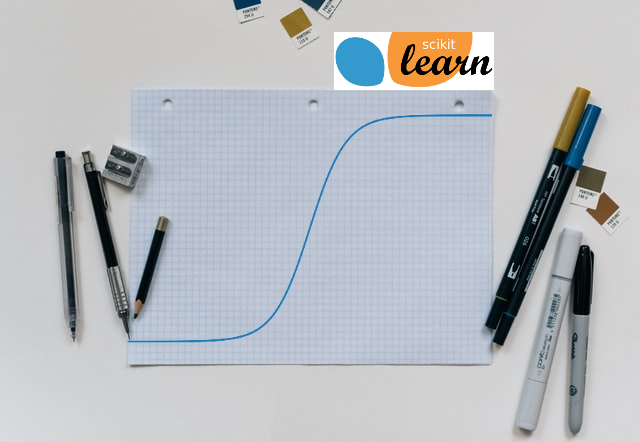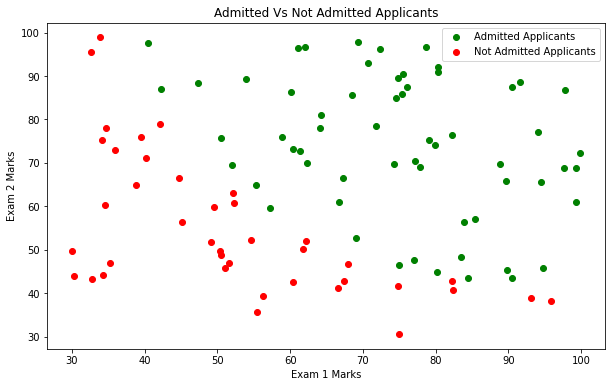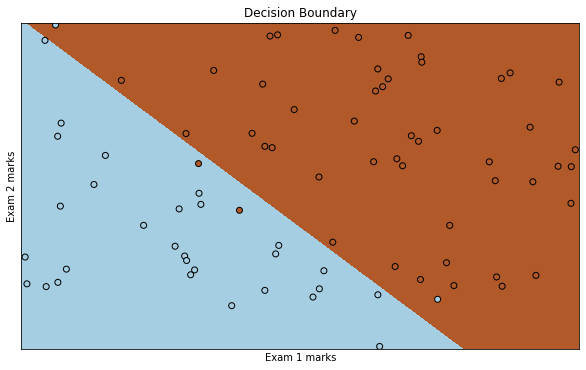# Binary Logistic Regression Using SklearnIn this tutorial we are going to use the Logistic Model from Sklearn library. We are also going to use the same test data used in Logistic Regression From Scratch With Python tutorial

## Introduction

Scikit-learn is one of the most popular open source machine learning library for python. It provides range of machine learning models, here we are going to use logistic regression linear model for classification. Sklearn logistic regression supports binary as well as multi class classification, in this study we are going to work on binary classification.

The way we have implemented our own cost function and used advanced optimization technique for cost function optimization in Logistic Regression From Scratch With Python tutorial, every sklearn algorithm also have cost function and optimization objective.

# Python Code

Now we will implement the Logistic regression algorithm in Python and build a classification model that estimates an applicant’s probability of admission based on Exam 1 and Exam 2 scores.

## Import Libraries

• pandas: Used for data manipulation and analysis
• numpy : Numpy is the core library for scientific computing in Python. It is used for working with arrays and matrices.
• matplotlib : It’s plotting library, and we are going to use it for data visualization
• model_selection: Here we are going to use train_test_split() class
• linear_model: Here we are going to LogisticRegression() class
``````import pandas as pd
import numpy as np
import matplotlib.pyplot as plt
from sklearn import model_selection
from sklearn import linear_model
``````

## Load The Data

• We are going to use ‘admission_basedon_exam_scores.csv’ CSV file
• File contains three columns Exam 1 marks, Exam 2 marks and Admission status
``````df = pd.read_csv('https://raw.githubusercontent.com/satishgunjal/datasets/master/admission_basedon_exam_scores.csv')
m, n = df.shape
print('Number of training examples = ', m)
print('Number of features = ', n - 1) # Not counting the 'Label: Admission status'
df.sample(5) # Show random 5 training examples
``````
``````Number of training examples =  100
Number of features =  2
``````
Exam 1 marks Exam 2 marks Admission status
26 80.190181 44.821629 1
76 47.264269 88.475865 1
25 62.271014 69.954458 1
81 94.834507 45.694307 1
91 90.448551 87.508792 1

## Data Understanding

• There are total 100 training examples (m= 100 or 100 no of rows)
• There are two features Exam 1 marks and Exam 2 marks
• Label column contains application status. Where ‘1’ means admitted and ‘0’ means not admitted

## Data Visualization

To plot the data of admitted and not admitted applicants, we need to first create separate data frame for each class(admitted/not-admitted)

``````df_admitted = df[df['Admission status'] == 1]
print('Dimension of df_admitted= ', df_admitted.shape)
df_admitted.sample(5)
``````
``````Dimension of df_admitted=  (60, 3)
``````
Exam 1 marks Exam 2 marks Admission status
96 42.261701 87.103851 1
83 57.238706 59.514282 1
85 68.468522 85.594307 1
59 71.796462 78.453562 1
88 78.635424 96.647427 1
``````df_notadmitted = df[df['Admission status'] == 0]
print('Dimension of df_notadmitted= ', df_notadmitted.shape)
df_notadmitted.sample(5)
``````
``````Dimension of df_notadmitted=  (40, 3)
``````
Exam 1 marks Exam 2 marks Admission status
29 38.785804 64.995681 0
64 44.668262 66.450086 0
35 54.635106 52.213886 0
92 55.482161 35.570703 0
61 35.286113 47.020514 0

Now lets plot the scatter plot for admitted and not admitted students

``````plt.figure(figsize = (10,6))
plt.scatter(df_admitted['Exam 1 marks'], df_admitted['Exam 2 marks'], color='green', label='Admitted Applicants')
plt.scatter(df_notadmitted['Exam 1 marks'], df_notadmitted['Exam 2 marks'], color='red', label='Not Admitted Applicants')
plt.xlabel('Exam 1 Marks')
plt.ylabel('Exam 2 Marks')
plt.legend()
plt.title('Admitted Vs Not Admitted Applicants')
``````## Create Feature Matrix X and Label Vector y

``````# Get feature columns from datframe
X = df.iloc[:, 0:2]
y = df.iloc[:, -1]
``````

## Create Test And Train Dataset

• We will split the dataset, so that we can use one set of data for training the model and one set of data for testing the model
• We will keep 20% of data for testing and 80% of data for training the model
• If you want to learn more about it, please refer Train Test Split tutorial
``````X_train, X_test, y_train, y_test = model_selection.train_test_split(X, y, test_size=0.2)

print('X_train dimension= ', X_train.shape)
print('X_test dimension= ', X_test.shape)
print('y_train dimension= ', y_train.shape)
print('y_train dimension= ', y_test.shape)
``````
``````X_train dimension=  (80, 2)
X_test dimension=  (20, 2)
y_train dimension=  (80,)
y_train dimension=  (20,)
``````

## Sklearn Logistic Regression

``````lm = linear_model.LogisticRegression()
# Training the model using training data
lm.fit(X_train, y_train)
``````
``````LogisticRegression(C=1.0, class_weight=None, dual=False, fit_intercept=True,
intercept_scaling=1, l1_ratio=None, max_iter=100,
multi_class='auto', n_jobs=None, penalty='l2',
random_state=None, solver='lbfgs', tol=0.0001, verbose=0,
warm_start=False)
``````

## Visualization

• Let’s plot decision boundary to cross-check the accuracy of our model
• I am using code from sklearns site. Reference
``````def plot_decision_boundary(X, Y, X_label, Y_label):
"""
Plot decision boundary based on results from sklearn logistic regression algorithm

I/P
----------
X : 2D array where each row represent the training example and each column represent the feature ndarray.
m= number of training examples
n= number of features
y : 1D array of labels/target value for each traing example.

O/P
-------
Plots the decision boundary
"""
logreg = linear_model.LogisticRegression(C=1e5)

# Create an instance of Logistic Regression Classifier and fit the data.
logreg.fit(X, Y)

# Plot the decision boundary. For that, we will assign a color to each
# point in the mesh [x_min, x_max]x[y_min, y_max].
x_min, x_max = X[:, 0].min() - .5, X[:, 0].max() + .5
y_min, y_max = X[:, 1].min() - .5, X[:, 1].max() + .5
h = .02  # step size in the mesh
xx, yy = np.meshgrid(np.arange(x_min, x_max, h), np.arange(y_min, y_max, h))
Z = logreg.predict(np.c_[xx.ravel(), yy.ravel()])

# Put the result into a color plot
Z = Z.reshape(xx.shape)
plt.figure(1, figsize=(10, 6))
plt.pcolormesh(xx, yy, Z, cmap=plt.cm.Paired)

# Plot also the training points
plt.scatter(X[:, 0], X[:, 1], c=Y, edgecolors='k', cmap=plt.cm.Paired)
plt.xlabel(X_label)
plt.ylabel(Y_label)

plt.xlim(xx.min(), xx.max())
plt.ylim(yy.min(), yy.max())
plt.xticks(())
plt.yticks(())

plt.title("Decision Boundary")
plt.show()
``````## Testing The Model

• For testing we are going to use the test data only
• Question: Predict an admission probability for applicant with scores 45 in Exam 1 and 85 in Exam 2
``````# Input data should be 2D array
prob = lm.predict_proba([[45, 85]])
print ('Probability of getting admission is %s, and probability of not getting admission is %s' % (prob[:,1], prob[:,0]))
``````
``````Probability of getting admission is [0.74012176], and probability of not getting admission is [0.25987824]
``````

## Accuracy Of Model

``````predictedAdmissionStatus= pd.DataFrame(lm.predict(X_test), columns=['Predicted Admission status'])
actualAdmissionStatus = pd.DataFrame(y_test, columns=['Admission status'])
actualAdmissionStatus = actualAdmissionStatus.reset_index(drop=True) # Drop the index so that we can concat it, to create new dataframe
df_actual_vs_predicted = pd.concat([actualAdmissionStatus,predictedAdmissionStatus],axis =1)
df_actual_vs_predicted.T
``````
0 1 2 3 4 5 6 7 8 9 10 11 12 13 14 15 16 17 18 19
Admission status 0 1 0 1 1 0 1 1 1 1 0 1 0 0 1 0 1 0 1 0
Predicted Admission status 1 1 0 1 1 1 1 1 1 1 0 1 0 0 1 1 1 0 0 0

## Probability For Entire Test Set

Probability output contains two columns, first column represent probability of negative class(0) and second column represent the probability of positive class(1)

``````lm.predict_proba(X_test)
``````
``````array([[9.76130141e-03, 9.90238699e-01],
[6.14312272e-02, 9.38568773e-01],
[8.15477257e-01, 1.84522743e-01],
[1.15938452e-05, 9.99988406e-01],
[6.45091832e-02, 9.35490817e-01],
[1.06897009e-01, 8.93102991e-01],
[1.35450967e-01, 8.64549033e-01],
[1.56234150e-04, 9.99843766e-01],
[5.62165345e-02, 9.43783465e-01],
[4.57353754e-03, 9.95426462e-01],
[9.99762159e-01, 2.37841324e-04],
[4.68658463e-06, 9.99995313e-01],
[9.93493276e-01, 6.50672430e-03],
[9.79207714e-01, 2.07922863e-02],
[1.05902317e-03, 9.98940977e-01],
[4.69621011e-03, 9.95303790e-01],
[3.11716254e-01, 6.88283746e-01],
[9.53870297e-01, 4.61297034e-02],
[6.91008637e-01, 3.08991363e-01],
[9.86840695e-01, 1.31593053e-02]])
``````

## Model Score

``````print('Model Score= ', lm.score(X_test, y_test))
``````
``````Model Score=  0.8
``````

## Conclusion

This concludes our binary logistic regression study using sklearn library. In next tutorial I will cover multi class logistic regression.

## Learning Path for DP-900 Microsoft Azure Data Fundamentals Certification

8 minute read

Learning path to gain necessary skills and to clear the Azure Data Fundamentals Certification. This certification is intended for candidates beginning to wor...

## Learning Path for AI-900 Microsoft Azure AI Fundamentals Certification

7 minute read

Learning path to gain necessary skills and to clear the Azure AI Fundamentals Certification. This certification is intended for candidates with both technica...

## ANN Model to Classify Images

12 minute read

In this guide we are going to create and train the neural network model to classify the clothing images. We will use TensorFlow deep learning framework along...

## Introduction to NLP

8 minute read

In short NLP is an AI technique used to do text analysis. Whenever we have lots of text data to analyze we can use NLP. Apart from text analysis, NLP also us...

## K Fold Cross Validation

14 minute read

There are multiple ways to split the data for model training and testing, in this article we are going to cover K Fold and Stratified K Fold cross validation...

## K-Means Clustering

13 minute read

K-Means clustering is most commonly used unsupervised learning algorithm to find groups in unlabeled data. Here K represents the number of groups or clusters...

## Time Series Analysis and Forecasting

10 minute read

Any data recorded with some fixed interval of time is called as time series data. This fixed interval can be hourly, daily, monthly or yearly. Objective of t...

## Support Vector Machines

9 minute read

Support vector machines is one of the most powerful ‘Black Box’ machine learning algorithm. It belongs to the family of supervised learning algorithm. Used t...

## Random Forest

12 minute read

Random forest is supervised learning algorithm and can be used to solve classification and regression problems. Unlike decision tree random forest fits multi...

## Decision Tree

13 minute read

Decision tree explained using classification and regression example. The objective of decision tree is to split the data in such a way that at the end we hav...

## Agile Scrum Framework

7 minute read

This tutorial covers basic Agile principles and use of Scrum framework in software development projects.

## Underfitting & Overfitting

2 minute read

Main objective of any machine learning model is to generalize the learning based on training data, so that it will be able to do predictions accurately on un...

## Multiclass Logistic Regression Using Sklearn

6 minute read

In this study we are going to use the Linear Model from Sklearn library to perform Multi class Logistic Regression. We are going to use handwritten digit’s d...

## Binary Logistic Regression Using Sklearn

5 minute read

In this tutorial we are going to use the Logistic Model from Sklearn library. We are also going to use the same test data used in Logistic Regression From Sc...

## Logistic Regression From Scratch With Python

13 minute read

This tutorial covers basic concepts of logistic regression. I will explain the process of creating a model right from hypothesis function to algorithm. We wi...

## Train Test Split

3 minute read

In this tutorial we are going to study about train, test data split. We will use sklearn library to do the data split.

## One Hot Encoding

11 minute read

In this tutorial we are going to study about One Hot Encoding. We will also use pandas and sklearn libraries to convert categorical data into numeric data.

## Multivariate Linear Regression Using Scikit Learn

8 minute read

In this tutorial we are going to use the Linear Models from Sklearn library. Scikit-learn is one of the most popular open source machine learning library for...

## Univariate Linear Regression Using Scikit Learn

7 minute read

In this tutorial we are going to use the Linear Models from Sklearn library. Scikit-learn is one of the most popular open source machine learning library for...

## Multivariate Linear Regression From Scratch With Python

10 minute read

In this tutorial we are going to cover linear regression with multiple input variables. We are going to use same model that we have created in Univariate Lin...

## Univariate Linear Regression From Scratch With Python

13 minute read

This tutorial covers basic concepts of linear regression. I will explain the process of creating a model right from hypothesis function to gradient descent a...

## Machine Learning Introduction And Learning Plan

4 minute read

In this tutorial we will see the brief introduction of Machine Learning and preferred learning plan for beginners

Back to top ↑